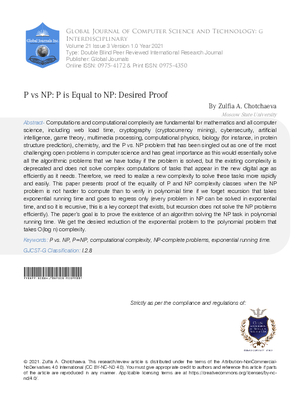### P vs NP: P is Equal to NP: Desired Proof

Authors

• Zulfia A. Chotchaeva

## DOI:

Keywords:

P vs NP, P=NP, computational complexity, NP-complete problems, exponential running time

Abstract

Computations and computational complexity are fundamental for mathematics and all computer science, including web load time, cryptography (cryptocurrency mining), cybersecurity, artificial intelligence, game theory, multimedia processing, computational physics, biology (for instance, in protein structure prediction), chemistry, and the P vs. NP problem that has been singled out as one of the most challenging open problems in computer science and has great importance as this would essentially solve all the algorithmic problems that we have today if the problem is solved, but the existing complexity is deprecated and does not solve complex computations of tasks that appear in the new digital age as efficiently as it needs. Therefore, we need to realize a new complexity to solve these tasks more rapidly and easily. This paper presents proof of the equality of P and NP complexity classes when the NP problem is not harder to compute than to verify in polynomial time if we forget recursion that takes exponential running time and goes to regress only (every problem in NP can be solved in exponential time, and so it is recursive, this is a key concept that exists, but recursion does not solve the NP problems efficiently). The papers goal is to prove the existence of an algorithm solving the NP task in polynomial running time. We get the desired reduction of the exponential problem to the polynomial problem that takes O(log n) complexity.

How to Cite

Zulfia A. Chotchaeva. (2021). P vs NP: P is Equal to NP: Desired Proof. Global Journal of Computer Science and Technology, 21(G3), 1–11. https://doi.org/10.34257/GJCSTGVOL21IS3PG1Submitted

2021-08-10

Published

2021-09-12

Issue

Section

Articles